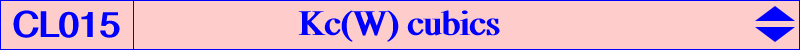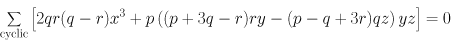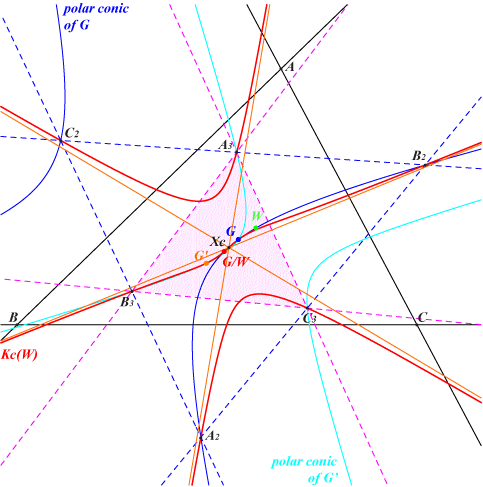Let W = (p : q : r) be a point. Kp(W) is the locus of pivots of all pK+ invariant under the isoconjugation with pole W. Kc(W) is the locus of the common point of the three asymptotes. Compare with the classes CL017 and CL018. Kp(W) and Kc(W) are always K+ cubics and, for a given W, they have the same asymptotic directions. When W = G, they degenerate into the union of the medians. Kc(W) is actually the homothetic image of Kp(W) under h(G/W, -1/3) where G/W = G-Ceva conjugate of W, the center of the circum-conic with perspector W. Both contain the (real or not) square roots Ro, Ra, Rb, Rc of W and G/W with a common tangent passing through G. Hence, Kp(W) and Kc(W) generate a pencil of cubics containing pK(W, G/W) and the degenerate cubic into the line at infinity and the diagonal conic passing through W and the square roots of W. The tangential of G/W in Kc(W) is G and the tangential of G/W in Kp(W) is aW/G, the homothetic of G/W under h(G,4). The asymptotes of Kp(W) concur at the reflection Xp of G about G/W and those of Kc(W) at the homothetic Xc of G/W under h(G,2/3). The tangents to Kp(W) at the four fixed points of the isoconjugation concur at W. This is false for Kc(W). Kp(W) and Kc(W) are K60+ cubics if and only if W = X(6). See Kp60 = K077 and Kc60 = K078. Kp(W) and Kc(W) are K++ cubics if and only if W lies on the Steiner inscribed ellipse but Kc(W)++ always degenerates. Kc(W) contains the following points : the fixed points Ro, Ra, Rb, Rc of the isoconjugation i.e. the square roots of W their homothetic images under h(G/W, -1/3) the homothetic of W/G. the centre of the conic isoconjugate of the line at infiny which is G/W. the centroid G. the infinite points of pK(W, G/W). See the figure in the page CL014. The barycentric equation of Kc(W) is :Kc(W) is a pivotal cubicThe polar conic of G meets Kc(W) at G (double), G/W and three other points A2, B2, C2. The polar conic of G' (tangential of G) meets Kc(W) at G' (double), G and three other points A3, B3, C3. The diagonal triangle of the quadrilateral A2, B2, C2, G is A3B3C3. Kc(W) is the pivotal cubic with pivot G and isopivot G' with respect to this triangle A3B3C3. Note that A2, B2, C2, G are the fixed points of the isoconjugation hence the tangents to Kc(W) at these points pass through the pivot G. Similarly, since A3, B3, C3 are the singular points of the isoconjugation, the tangents at these points and at the pivot G pass through the isopivot G'.